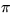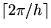Next: domain Up: Reference guide: Previous: blink

### circle

NAME

circle

SYNOPSIS

          circle x0 y0 r [-optional link arguments]


DESCRIPTION

circle creates a circular entity of immersed boundary points. circle requires three arguments.
• x0 - x coordinate of the circle center
• y0 - y coordinate of the circle center
• r - the radius of the circle
circle allows for optional link arguments by interpreting the following options.
• -A name - indicates this entity is active and provides a name
• -a angle - resting angle at the IB points
• -b bend - bending stiffness at the IB points (default=40000)
• -d distance - initial distance between IB points (default=0.5)
• -N name - provides a name for the entity
• -n number - number of IB points around the circle
• -s stiff - inter-IB-point spring stiffness (default=40000)
• -t tether - tether spring stiffness (default=0)
The default resting length is determined so the points are no more than a distance of h/2 apart. The default resting angle is determined so that the circle remains a circle if there is no fluid flow. The tether points are located at the initial IB point positions.

line, polygon, wheel

EXAMPLE

          circle 1.0 1.25 0.5 -b 10000

Forms a circle centered at (1,1.25) with a radius of 0.5 and increases the bending stiffness to 10000. The circumference of the circle is, and the resting length is not specified so the number of immersed boundary points set by IBIS isand they are equally spaced on the circle.Next: domain Up: Reference guide: Previous: blink
David Eyre
6/19/1998